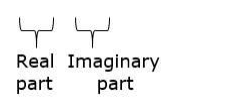# Simplify each of the following and express it in the form (a + ib) :

Question:

Simplify each of the following and express it in the form (a + ib) :

$(2+\sqrt{-3})^{2}$

Solution:

Given: $(2-\sqrt{-3})^{2}$

We know that

$(a-b)^{2}=a^{2}+b^{2}-2 a b \ldots(i)$

So, on replacing a by 2 and b by $\sqrt{-3}$ in eq. (i), we get

$(2)^{2}+(\sqrt{-3})^{2}-2(2)(\sqrt{-3})$

$=4+(-3)-4 \sqrt{-3}$

$=4-3-4 \sqrt{-3}$

$=1-4 \sqrt{3} \mathrm{i}^{2}\left[\because \mathrm{i}^{2}=-1\right]$

$=1-4 \mathrm{i} \sqrt{3}$